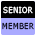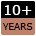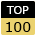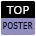Welcome to WebmasterWorld Guest from 35.172.195.49

Forum Moderators: coopster & jatar k

Message Too Old, No Replies

# Finding out which combination of numbers equals \$x

#### jecasc

5:12 pm on Jan 8, 2011 (gmt 0)

#### Senior Member from DEjoined:May 8, 2003
posts:1144

I have the following problem:

I have a variable \$x for example: 2138.25

I have several numbers in an array \$numbers[]:
123.23
348.56
1435.33
702.92
744.95
...

Now I need to find out, which combination of the numbers equal \$x. Problem is: How many of the given numbers equal \$x is also a variable. Sometimes its a combination of two numbers, sometimes its three or four, or five.

So basically I have:
\$x = a given sum
\$numbers[] = an array of numbers where \$y of them equal \$x
\$y = how many of numbers[] are needed to equal \$x

Anybody could point me in the right direction on how to do this in PHP?

#### jecasc

5:32 pm on Jan 8, 2011 (gmt 0)

#### Senior Member from DEjoined:May 8, 2003
posts:1144

[offtopic]Wow, Google has become fast. I searched for a solution in Google just now, and was delighted when it showed up a Search Result with exactly the question I had asked here a few moments ago. I clicked on the result and - ended up here. It was my own question already showing in the SERPS.[/offtopic]

#### Frank_Rizzo

9:36 pm on Jan 8, 2011 (gmt 0)

#### Senior Memberjoined:June 17, 2002
posts:1189

At first I thought you would need to traverse pascal's triangle until a match is met. This will solve if any one number, or any two numbers .... any (arraylength) numbers equal \$x

But I don't understand

\$numbers[] = an array of numbers where \$y of them equal \$x
\$y = how many of numbers[] are needed to equal \$x

#### jecasc

10:28 pm on Jan 8, 2011 (gmt 0)

#### Senior Member from DEjoined:May 8, 2003
posts:1144

What I mean is this for example:

numbers[] = array(4,8,2,35,67)
y=2 would mean that the sum of two elements in numbers[] equal the end result, y=4 would mean the sum of four elements of numbers[] is needed.

#### jecasc

9:34 am on Jan 10, 2011 (gmt 0)

#### Senior Member from DEjoined:May 8, 2003
posts:1144

Gin!

Don't know if getting drunk will help me find a solution. But I'll try anyway. Thanks for the suggestion.

#### tangor

9:47 am on Jan 10, 2011 (gmt 0)

#### Senior Member from USjoined:Nov 29, 2005
posts:10574

It is obvious you should not play Gin Rummy with me, jecac! But getting drunk from time to time might bring inspiration (most of which will be "Why did I do that?" and more importantly "What problem am I trying to solve?"

Your array and query make no sense TO ME. Need a bit more. And I am a number cruncher, hence the humorous "Gin!" remark.

#### penders

10:58 am on Jan 10, 2011 (gmt 0)

#### Senior Memberjoined:July 3, 2006
posts: 3153

Once the hangover clears...

Do you expect only 1 solution with your data? What if there are multiple? eg.
`\$NUMBERS = array (1,2,3,4,5,6,7,8,9,10); \$TARGET = 10; // Solutions: 10, 8+2, 7+3, 6+4, 7+2+1, ...`

#### jecasc

12:30 pm on Jan 10, 2011 (gmt 0)

#### Senior Member from DEjoined:May 8, 2003
posts:1144

Do you expect only 1 solution with your data? What if there are multiple?

If there are multiple solutions it would be best if I get all of them:
For example if I have:

\$NUMBERS = array (5,23,43,411,9,11,34,87,4,12);
\$TARGET = 39
\$y = 3

Then I would expect the solutions:
5,11,23 and 4,12,23

For:
\$NUMBERS = array (52,231,43,93,411,94,11,334,827,12);
\$TARGET = 200
\$y = 4
I would expect: 52,43,94,11 and 52,43,93,12

I know how I could do this if \$y where a fixed value, for example \$y = 2. Then I simply would use two nested "for" loops and try all combinations. However I don't know how to do it if \$y is a variable.

#### penders

1:09 pm on Jan 10, 2011 (gmt 0)

#### Senior Memberjoined:July 3, 2006
posts: 3153

Ah, is \$y a value you give to the function? ie. I want a solution with 4 values (\$y = 4)?

Or (this is what I was assuming)... \$y is returned from the function, indicating the number of values in the solution (although this is simply a count of the numbers in the solution and doesn't need to be calculated separately).

#### jecasc

2:00 pm on Jan 10, 2011 (gmt 0)

#### Senior Member from DEjoined:May 8, 2003
posts:1144

Ah, is \$y a value you give to the function? ie. I want a solution with 4 values (\$y = 4)?

Yes, \$y is not returned but a given variable. So \$y=2 means I want to know which two numbers in \$NUMBERS equal \$TARGET, if \$y=4 I want to know which four numbers in \$NUMBERS equal \$TARGET. Not up to four numbers but exactly four.

#### Frank_Rizzo

3:48 pm on Jan 10, 2011 (gmt 0)

#### Senior Memberjoined:June 17, 2002
posts:1189

How large can \$y be?

---

Rather than a card game this is more like lottery or horse racing combinations.

#### azazello

4:03 pm on Jan 10, 2011 (gmt 0)

#### New Userjoined:Apr 16, 2006
posts:15

How big can your set grow?

As the size of your set grows the more difficult the problem becomes.

Take a look at :-

[stackoverflow.com...]

[en.wikipedia.org...]

#### jecasc

7:13 pm on Jan 10, 2011 (gmt 0)

#### Senior Member from DEjoined:May 8, 2003
posts:1144

How large can \$y be?

I guess that \$y would normally be between 2 and 8 and normally there should not be more than 10 elements in \$NUMBERS. Maybe I'll just write one function for \$y=2, one for \$y=3 and so on. This should be relatively easy to do. If \$y is much bigger than 8 it will take too much time to process anyway.

#### Hire Expert Members